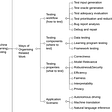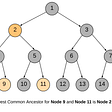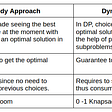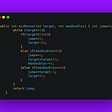# Ternary Search Convex Optimization

One of the fundamental problems we encounter as ML scientist/engineer is that of optimization. Most ML problems require optimization of a cost function.

Assuming that we have a convex loss function, which is mostly the case with mean squared error loss function. We are tasked with minimizing the loss i.e. find the parameters of a function where the loss is minimum.

In general the problem is multi-dimensional i.e. the input (feature space) has multiple dimensions or variables. ML engineers love to use their favourite “gradient descent” tool to solve such optimization problems. But often “gradient descent” is a sub-optimal approach as it can lead to too many iterations to find an optimal solution.

Given a simplification of a 1D convex optimization function F(x) and also the domain of the function [a, b] i.e. the function F is defined for a ≤ x ≤ b. Find ‘x’ where F(x) is minimum.

Can we use Binary Search to solve this problem ?

Let us say that the optimum x is x0 i.e. F(x0) is minimum. It implies that F decreases from a to x0 and increases from x0 to b i.e. F(a) > F(x0) < F(b).

Let us take some middle value between a and b, call it c = (a+b)/2.

Now F(a) > F(c) < F(b), but c may not be the optimum value.

But the thing is that c can be either between a and x0 or between x0 and b so we do not know which direction to move with just c.

One way we can overcome this situation is to consider another c’=c+delta where delta is a very small positive real number such as 1e-9.

If F(c’) < F(c) it implies c is between a and x0 and we can do binary search between c and b.

If F(c’) > F(c) it implies c is between x0 and b and we can do binary search between a and c.

Assuming F(x) = (x-2)², where the minimum occurs at x=2.

But note that this solution assumes that F is continuous as it allows us to find F(x) for any arbitrary real valued x.

But it may be that we do not the functional form of F(x) and F is simply an array of values which is decreasing initially then increasing.

F = [10, 9, 6, 1, 3, 27, 34, 97, 123, 1000]

Another solution is to use Ternary Search.

What if instead of one c, we have c1 and c2 between a and b i.e. c1 < c2 and F(a) > F(c1) < F(b) and F(a) > F(c2) < F(b).

If F(c1) > F(c2), it implies that c1 must be between a and x0 and we can reduce our search space between c1 and b.

If F(c1) < F(c2), it implies that c2 must be between x0 and b and we can reduce our search space between a and c2.

Else if F(c1) = F(c2), then it implies that c1 and c2 are on the opposite sides of x0 if c1 != c2 and we reduce search space to [c1, c2], else if c1 = c2 then it is the minimum.

To select c1 and c2, we can divide the space between a and b into 3 equal parts, and put c1 at a+(b-a)/3 and c2 at a+2*(b-a)/3.

‘delta’ controls the precision of our result.

Time complexity of both binary search and ternary search is O(logN) where N = (b-a).

https://cp-algorithms.com/num_methods/ternary_search.html

--

--

--

## More from Abhijit Mondal

Engineer

Love podcasts or audiobooks? Learn on the go with our new app.

## ML System Design — ETA Prediction## ML Model Testing## Natural Language Tool Kit 3.5## Why ReLU is not used in output layer of neural net?## Why Logistic regression is called regression despite being used for binary classification problem?## Numerical Method Considerations for Machine Learning## Preparing for the GCP Machine Learning Certification## Trying to make sense of EconMLEngineer

## DFS recursive solution to find Lowest Common Ancestor (LCA) of a Binary Tree## DS & Algo Problems — Rotate or Modify Array in Place## Greedy Algorithms## Minimum Moves to Reach Target Score